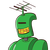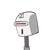# If 15 cans hold 135 litres of milk , how many such cans will be needed to store 180 litres of milk​

If 15 cans hold 135 litres of milk , how many such cans will be needed to store 180 litres of milk​

### 2 thoughts on “If 15 cans hold 135 litres of milk , how many such cans will be needed to store 180 litres of milk​”

1.20 cans

Step-by-step explanation:

since, 135 litres hold by 15 cans

therefore 1 litre hold by 15/135

therefore 180 litre hold by 1/9*180

therefore 20 cans needed

2.⇒ Given:

15 cans can hold 135 litres of milk.

⇒ To Find:

The no. of cans that are needed to store 180 litres of milk.

⇒ Solution:

In order to solve this question, we will be using the concept of unitary method where all the measures are found out in their singular form and then multiplied to the required measures as per the question.

Here, it is given that 15 cans can hold 135 litres of milk. To move forward, we will have to to find the amount of milk a single can can hold.$$\sf{\longrightarrow\:Capacity\:of\:1\:can=\dfrac{Total\:milk\:held\:by\:total\:cans}{Total\:cans}}$$

$$\sf{\longrightarrow\:Capacity\:of\:one\:can=\dfrac{135}{15}}$$

$$\sf{\longrightarrow\:Capacity\:of\:one\:can=\dfrac{27}{3}}$$

$$\bf{\longrightarrow\:Capacity\:of\:one\:can=9\:litres}$$

Now, we know that a single can has a capacity of 9 litres. Here, it is asked to find the no. of cans which can be found as follows:

$$\sf{\longrightarrow\:No.\:of\:cans=\dfrac{Total\:litres}{Capacity\:of\:1\:can}}$$

$$\sf{\longrightarrow\:No.\:of\:cans=\dfrac{180}{9}}$$

$$\bf{\longrightarrow\:No.\:of\:cans=20}$$

Therefore 20 cans are required to hold 180 litres of milk.

### Unitary Method:

By applying the unitary method, we find the measure of one single unit and apply that measure to find the total value of the units as per the question provided. Unitary method is used in a wide variety of mathematical branches including ratios. There are two types of results of unitary method:

✳ Direct variation

As the unit becomes singular (decreased), the value also decreases.

✳ Indirect variation

When either of the two terms is in an increase, the other term is on a decrease.